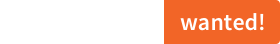sciss / kollflitzUseful methods for Scala collections.

KollFlitz

Statement

KollFlitz provides extension methods for the Scala standard collection library. Things that I tend to require often, like calculating mean, variance or percentiles, mapping groups, counting occurrences etc., as well as a number of collection operations available in SuperCollider.

It is released under the GNU Lesser General Public License v2.1+ and comes with absolutely no warranties. To contact the author, send an email to contact at sciss.de.

Note

This is used for scientific projects and rapid prototyping. The emphasis is not on performance optimisation.

Requirements / Installation

This project builds with sbt against Scala 2.13, 2.12 (JVM), and Scala 2.13 (JS). The last version to support Scala 2.11 was v0.2.3.

To use the library in your project:

"de.sciss" %% "kollflitz" % v

The current version v is "0.2.4".

To play around with these operations, run sbt console.

Operations

On any type of collection, sequential or not (Iterable)

method description example
counted creates a map from elements to the frequencies of their occurrence. List(13, 5, 8, 21, 3, 8).counted == Map(3 -> 1, 5 -> 1, 8 -> 2, 13 -> 1, 21 -> 1)
meanVariance returns a tuple of mean and variance, using an implicit Fractional type class. List(13.0, 5.0, 9.0).meanVariance == (9.0, 32.0)
mean returns the mean value, using an implicit Fractional type class. List(13.0, 5.0, 9.0).mean == 9.0
variance returns the variance value, using an implicit Fractional type class. List(13.0, 5.0, 9.0).variance == 5.0
normalized transforms the elements by dividing them by the maximum absolute value. List(13.0, 5.0, -9.0).normalized == List(1.0, 0.3846, -0.6923)
toMultiMap takes a key and a value view function and produces a multi-map. List("a1", "b1", "a2", "b3", "c2", "c3").toMultiMap(_.head)(_.drop(1).toInt) == Map(b -> Vector(1, 3), a -> Vector(1, 2), c -> Vector(2, 3))
allDistinct determines whether all elements are unique. List(1, 2, 3).allDistinct == true && List(1, 2, 1).allDistinct == false

On sequential collections (SeqLike)

method description example
groupWith groups the sequence such that within the adjacent elements of each group a predicate holds. List(13, 5, 8, 21, 3, 8).groupWith(_ > _).toVector == Vector(List(13, 5), List(8), List(21, 3), List(8))
isSorted determines whether the collection is sorted are boolean tests. List(1, 2, 3).isSorted == true && List(1, 2, 1).isSorted == false
isSortedBy determines whether the collection is sorted (using a mapping function) List(3, 2, 1).isSortedBy(-_) == true
mapPairs a mapping operation taking a function with two arguments which is applied with adjacent elements. List(13, 5, 8, 21, 3, 8).mapPairs(_ - _) == List(8, -3, -13, 18, -5)
foreachPairs iterates using a function with two arguments which is applied with adjacent elements. foreachPairs is to mapPairs what foreach is to map.
differentiate is a special pair map that uses a numeric type class to calculate the pairwise differences. List(13, 5, 8, 21, 3, 8).differentiate == List(-8, 3, 13, -18, 5)
integrate the opposite of differentiate. It aggregates a running sum. List(13, -8, 3, 13, -18, 5).integrate == List(13, 5, 8, 21, 3, 8)
stutter repeats each element of the input collection a number of times. List(13, -8, 3).stutter(2) == List(13, 13, -8, -8, 3, 3)
mirror concatenates the collection with the tail of its reverse sequence. List(13, -8, 3).mirror == List(13, -8, 3, -8, 13)
decimate drops all but one element from each n elements. List(13, 5, 8, 21, 3, 8).decimate(2) == List(13, 8, 3)
wrapAt returns an element, wrapping the index around the boundaries List(13, 5, 8, 21).wrapAt(-1) == 21
foldAt returns an element, folding (mirroring) the index within the boundaries List(13, 5, 8, 21).foldAt(-1) == 5
clipAt returns an element, clipping the index to the boundaries List(13, 5, 8, 21).clipAt(-1) == 13
minIndex determines the index of the minimum element List(13, 5, 8, 21).minIndex == 1
maxIndex determines the index of the maximum element List(13, 5, 8, 21).maxIndex == 3
minIndexBy determines the index of the minimum element, based on a mapping function List(13, 5, 8, 21).minIndexBy(-_) == 3
maxIndexBy determines the index of the maximum element, based on a mapping function List(13, 5, 8, 21).maxIndexBy(-_) == 1

sortedT, sortByT, sortedWithT are just forwarders, but they tag the result with type Sorted to prevent mistakes when calling methods such as percentile which assume that the collection is already sorted. Thus you can write List(1, 3, 2, 7).sortedT.median but not List(1, 3, 2, 7).sorted.median.

On sorted indexed collections (IndexedSeq @@ Sorted)

method description example
percentile returns a given percentile of the sorted collection. It picks an integer index and does not interpolate
values. Vector(13, 5, 8, 21, 3, 8).sortedT.percentile(75) == 13
median a shortcut for percentile(50)

Random operations

Random operation require an implicit value of type scala.util.Random. They must be imported via RandomOps._.

method description
choose returns a random element of a sequence.
shuffle returns a copy of the collection where the order of elements is randomised.
toUrn returns a scrambled iterator (an argument specifies whether it is infinite or not)

Limitations

The acrobatics for allowing String and Array to be extended by these operations have not been performed.